Courses

# Test: Problems On Ages - 1

## 10 Questions MCQ Test General Aptitude for GATE 2020 | Test: Problems On Ages - 1

Description
This mock test of Test: Problems On Ages - 1 for Banking Exams helps you for every Banking Exams entrance exam. This contains 10 Multiple Choice Questions for Banking Exams Test: Problems On Ages - 1 (mcq) to study with solutions a complete question bank. The solved questions answers in this Test: Problems On Ages - 1 quiz give you a good mix of easy questions and tough questions. Banking Exams students definitely take this Test: Problems On Ages - 1 exercise for a better result in the exam. You can find other Test: Problems On Ages - 1 extra questions, long questions & short questions for Banking Exams on EduRev as well by searching above.
QUESTION: 1

### Ten years ago, P was half of Q's age. If the ratio of their present ages is 3:4, what will be the total of their present ages?

Solution:

Let present age of P and Q be 3x
and  4x  respectively.
Ten years ago, P was half of Q's age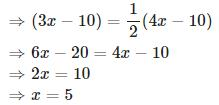Total of their present ages
=3x+4x=7x=7×5=35

QUESTION: 2

### Father is aged three times more than his son Sunil. After 8 years, he would be two and a half times of Sunil's age. After further 8 years, how many times would he be of Sunil's age?

Solution:

Assume that Sunil's present age
= x.
Then, father's present age  =3x + x = 4x

After 8 years, father's age =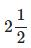times of Sunils' age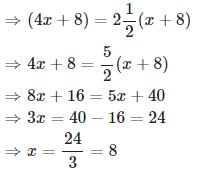After further  8  years,
Sunil's age  = x + 8 + 8 = 8 + 8 + 8 = 24
Father's age  = 4 x + 8 + 8 = 4 x 8 + 8 + 8 = 48

Father's age/Sunil's age = 48/24 = 2

QUESTION: 3

### A man's age is 125%of what it was 10 years ago, but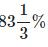of what it will be after 10years. What is his present age?

Solution:

Let the age before 10 years =x. Then,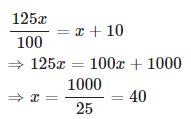Present age = x+10=40+10=50

QUESTION: 4

A man is 24 years older than his son. In two years, his age will be twice the age of his son. What is the present age of his son?

Solution:

Let present age of the son = x years
Then, present age the man = ( x + 24 ) years

Given that, in 2 years, man's age will be twice the age of his son
⇒(x+24)+2=2(x+2)
⇒x=22

QUESTION: 5

Present ages of Kiran and Syam are in the ratio of 5 : 4 respectively. Three years hence, the ratio of their ages will become 11 : 9 respectively. What is Syam's present age in years?

Solution:

Ratio of the present age of Kiran and Syam = 5 : 4

Let present age of Kiran = 5x
Present age of Syam = 4x
After 3 years, ratio of their ages = 11 : 9
⇒ ( 5 x + 3 ) : ( 4 x + 3 ) = 11 : 9
⇒ 9 ( 5 x + 3 ) = 11 ( 4 x + 3 )
⇒ 45 x + 27 = 44 x + 33
⇒ x = 33 − 27 = 6
Syam's present age = 4 x = 4 × 6 = 24

QUESTION: 6

The sum of ages of 5 children born at the intervals of 3 years each is 50 years. Find out the age of the youngest child?

Solution:

Let the age of the youngest child = x
Then, the ages of 5 children can be written as x , ( x + 3 ) , ( x + 6 ) , ( x + 9 ) and ( x + 12 )
x + ( x + 3 ) + ( x + 6 ) + ( x + 9 ) + ( x + 12 ) = 50
⇒ 5 x + 30 = 50
⇒ 5 x = 20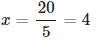QUESTION: 7

A is two years older than B who is twice as old as C. The total of the ages of A, B and C is 27 . How old is B?

Solution:

Let age of C = x .

Then, Age of B = 2x
Age of A = 2 + 2x
Total age of A,B and C =27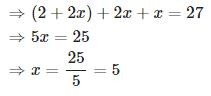B's age =2x=2×5=10

QUESTION: 8

The average age of a class of 22 students is 21 years. The average increased by 1 when the teacher's age also included. What is the age of the teacher?

Solution:

Total age of all students
= 22 × 21

Total age of all students + Age of the teacher  = 23 × 22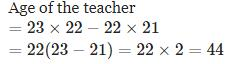QUESTION: 9

A father said to his son, "I was as old as you are at the present at the time of your birth". If the father's age is 38 years now, what was the son's age five years back?

Solution:

Let son's present age be x years. Then,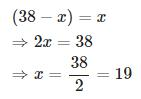Son's age 5  years back = 19 − 5 = 14

QUESTION: 10

Ayisha's age is 1 / 6 th of her father's age. Ayisha's father's age will be twice Shankar's age after 10 years. If Shankar's eight birthdays was celebrated two years before, then what is Ayisha's present age.

Solution:

Let Ayisha's present age = x
Then, her father's age = 6 x
Given that Ayisha's father's age will be twice Shankar's age after 10 years. Therefore, Shankar's age after 10 years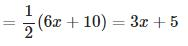Also given that Shankar's eight birthdays was celebrated two years before. Therefore, Shankar's age after 10 years = 8 + 12 = 20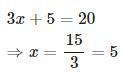Therefore, Ayisha's present age =5 years